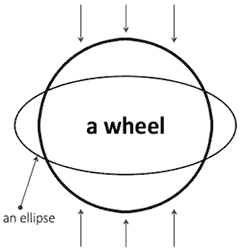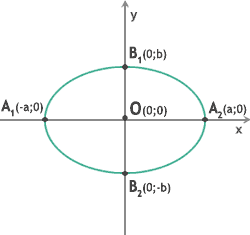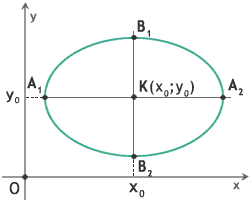# What Is an Ellipse

If you don't know what is an ellipse you should imagine a wheel (e.g. bike wheel). It is blown off evenly.

Look at the picture!The wheel is unusable now but on the other hand we have a perfect ellipse. Our life helps us to know math!

So, what is an ellipse in math? An ellipse is a geometric figure. You can get it in the geometric plane.

Here is the canonical equation of the ellipse:

x2a2
+
y2b2
=1 ,
a, b ∈ R   a>0; b>0; a>b

## Points:O(0;0) - the centre of the ellipse A1(-a;0) - the tops of the ellipse A2(a;0) B1(0;b) B2(0;-b) |A1A2| = 2a - the major axis of the ellipse |B1B2| = 2b - the minor axis of the ellipse |OA1| = |OA2| = a - the semimajor axis of the ellipse |OB1| = |OB2| = b - the semiminor axis of the ellipse

So, the canonical equation of the ellipse with the centre (0;0) and the semimajor axis a, the semiminor axis b is:

x2a2
+
y2b2
=1

If you need an ellipse with the centre in the point K(xo;yo), then you should use the equation:

(x - xo)2a2
+
(y - yo)2b2
=1

|KA1| = |KA2| = a - the semimajor axis of the ellipse

|KB1| = |KB2| = b - the semiminor axis of the ellipseCalculators